QuotientCustom SearchQUOTIENT In this section three methods of integrating quotients are discussed, but only the second method will be proven. The first method is to put the quotient into the form of the power of a function. The second method results in operations with logarithms. The third method is a special case in which the quotient must be simplified to use the sum rule. METHOD 1 If we are given the integralwe observe that this integral may be written asBy letting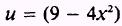thenThe only requirement for this to fit the form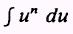is the factor for du of - 4. We accomplish this by multiplying 2x dx by - 4, giving - 8x dx, which is du. We then compensate for the factor - 4 by multiplying the integral by -1 /4. Therefore,EXAMPLE: Evaluate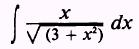SOLUTION:Letso thatThe factor 2 is used in the integral to give du and is compensated for by multiplying the integral by 1/2. Therefore,PRACTICE PROBLEMS: Evaluate the following integrals:ANSWERS: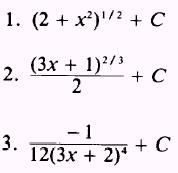METHOD 2 In the previous formulas for integration of a function, the exŁponent was not allowed to be -1. In the special case of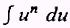where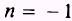we would have applied the following formula: Formula.Integrated Publishing, Inc. - A (SDVOSB) Service Disabled Veteran Owned Small Business